Multiplication of Numbers with 3 Digits
 What is a Multiplication of Numbers with 3 Digits? Standard Method  Abyssinian Farmers' Multiplication Grid Method Cross Method  Strip Method Multiplication on the Internet References.
 To the Main Page  "Mathematische Basteleien"

What is a Multiplication of Numbers with 3 Digits?  top
 ...... If you must multiply numbers with several digits, you must use the "written" multiplication as we say in Germany.  There are various methods of multiplication on this website with 896x271 as an example.

Standard Method in Germany top
 1) Problem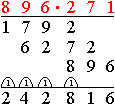2) Multiply 3x3) Add 6x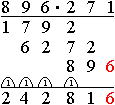4) Result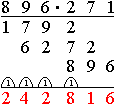Background: 896*271 = (800+90+6)*271 =  800*271+90*271+6*271

Variations of this method
 Detailed, for beginners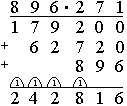In the past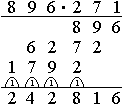rare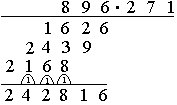English Method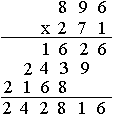Abyssinian Farmers' Multiplication    top
 1) Halve 9x without remainder and double.9x.2) Delete the lines with even numbers in the left-hand column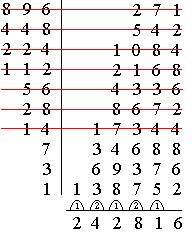3) Add 6x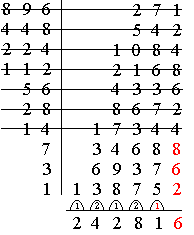4) ResultLines with even numbers are deleted, because the Abyssinian Farmers think: "Even numbers give bad luck."
This method is also called Russian farmers' method.

Background:  896*271 = (512+256+128)*271 = (2^9+2^8+2^7)*271 = 2^9*271+2^8*271+2^7*271  = 34688+69376+138752 = 242816.
The first factor becomes a binary number. - The multiplication is reduced to halving and doubling.

Neper's Grid method  top
 1) Problem2) Multiply 9x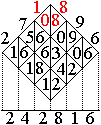3) Add 6x4) ResultAnother shape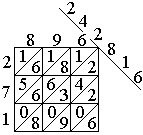Background: 896*271 = (800+90+6)*(200+70+1)=8*2*10.000+8*7*1000+8*1*100 + 9*2*1000+9*7*100+9*1*10 + 6*2*100+6*7*10+6*1.

Cross Method    top
 Draw the following picture and give a figure to each part.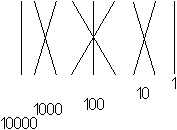Write corresponding digits on the ends of the lines.  The product is  896 *271.Form terms of the numbers and calculate.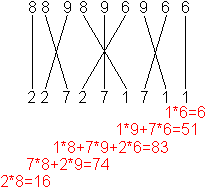Keep the results in your head. Write down the results and take into account the amount carried over.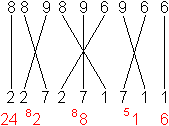Write down this picture only in this method.

Background: 896*271 = (800+90+6)*(200+70+1)=8*2*10.000+8*7*1000+8*1*100 + 9*2*1000+9*7*100+9*1*10 + 6*2*100+6*7*10+6*1.

Peter Müller from Saarbrücken sent me this method. Thanks.

Strip method     top
Write the second factor on a strip of paper, move the strip to the left, multiply and add.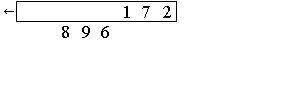Overall view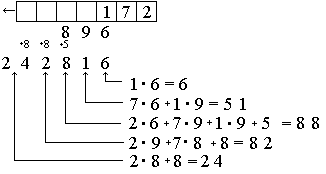Background: 896*271 = (800+90+6)*(200+70+1)
= 8*2*10,000+8*7*1000+8*1*100 + 9*2*1000+9*7*100+9*1*10 + 6*2*100+6*7*10+6*1.

Multiplication on the Internet      top

German

Wikipedia
Multiplikation, Russische Bauernmultiplikation

English

Alexander Bogomolny (Cut The Knot)
Peasant MultiplicationLong Multiplication

References  top
Ewald Fettweis: Wie man einstens rechnete, Leipzig 1923
Walter Sperling: Auf du und du mit Zahlen, Rüschlikon-Zürich 1955

Feedback: Email address on my main page